# Periodic boundary value problems for second-order impulsive integro-differential equations with integral jump conditions

## Abstract

This paper is concerned with the existence of extremal solutions of periodic boundary value problems for second-order impulsive integro-differential equations with integral jump conditions. We introduce a new definition of lower and upper solutions with integral jump conditions and prove some new maximum principles. The method of lower and upper solutions and the monotone iterative technique are used.

MSC: 34B37, 34K10, 34K45.

## 1 Introduction

Differential equations which have impulse effects describe many evolution processes that abruptly change their state at a certain moment. In recent years, impulsive differential equations have become more important tools in some mathematical models of real processes and phenomena studied in physics, biotechnology, chemical technology, population dynamics and economics; see . Many papers have been published about existence analysis of periodic boundary value problems of first and second order for impulsive ordinary or functional or integro-differential equations. We refer the readers to the papers . More recent works on existence results of impulsive problems with integral boundary conditions can be found in  and the reference therein. This literature has lead to significant development of a general theory for impulsive differential equations.

The monotone iterative technique coupled with the method of upper and lower solutions has been used to study the existence of extremal solutions of periodic boundary value problems for second-order impulsive equations; see, for example, . This method has been also used to study abstract nonlinear problems; see . However, in most of these papers concerned with applications of the monotone iterative technique to second-order periodic boundary value problems with impulses, the authors assume that the jump conditions at impulse point ${t}_{k}$ of solution values and the derivative of solution values depend on the left-hand limits of solutions or the slope of solutions themselves, such as $\mathrm{\Delta }x\left({t}_{k}\right)={I}_{k}\left(x\left({t}_{k}^{-}\right)\right)$, $\mathrm{\Delta }x\left({t}_{k}\right)={I}_{k}\left({x}^{\prime }\left({t}_{k}^{-}\right)\right)$, $\mathrm{\Delta }{x}^{\prime }\left({t}_{k}\right)={I}_{k}\left(x\left({t}_{k}^{-}\right)\right)$, $\mathrm{\Delta }{x}^{\prime }\left({t}_{k}\right)={I}_{k}\left({x}^{\prime }\left({t}_{k}^{-}\right)\right)$.

In this paper, we consider the periodic boundary value problem for second-order impulsive integro-differential equation (PBVP) with integral jump conditions:

$\left\{\begin{array}{c}{x}^{″}\left(t\right)=f\left(t,x\left(t\right),\left(Kx\right)\left(t\right),\left(Sx\right)\left(t\right)\right),\phantom{\rule{1em}{0ex}}t\in J=\left[0,T\right],t\ne {t}_{k},\hfill \\ \mathrm{\Delta }x\left({t}_{k}\right)={I}_{k}\left({\int }_{{t}_{k}-{\delta }_{k}}^{{t}_{k}-{\epsilon }_{k}}{x}^{\prime }\left(s\right)\phantom{\rule{0.2em}{0ex}}ds\right),\phantom{\rule{1em}{0ex}}k=1,2,\dots ,m,\hfill \\ \mathrm{\Delta }{x}^{\prime }\left({t}_{k}\right)={I}_{k}^{\ast }\left({\int }_{{t}_{k}-{\tau }_{k}}^{{t}_{k}-{\sigma }_{k}}x\left(s\right)\phantom{\rule{0.2em}{0ex}}ds\right),\phantom{\rule{1em}{0ex}}k=1,2,\dots ,m,\hfill \\ x\left(0\right)=x\left(T\right),\phantom{\rule{2em}{0ex}}{x}^{\prime }\left(0\right)={x}^{\prime }\left(T\right),\hfill \end{array}$
(1.1)

where $0={t}_{0}<{t}_{1}<{t}_{2}<\cdots <{t}_{k}<\cdots <{t}_{m}<{t}_{m+1}=T$, $f:J×{R}^{3}\to R$ is continuous everywhere except at $\left\{{t}_{k}\right\}×{R}^{3}$, $f\left({t}_{k}^{+},x,y,z\right)$, $f\left({t}_{k}^{-},x,y,z\right)$ exist, $f\left({t}_{k}^{-},x,y,z\right)=f\left({t}_{k},x,y,z\right)$, ${I}_{k}\in C\left(R,R\right)$, ${I}_{k}^{\ast }\in C\left(R,R\right)$, $\mathrm{\Delta }x\left({t}_{k}\right)=x\left({t}_{k}^{+}\right)-x\left({t}_{k}^{-}\right)$, $\mathrm{\Delta }{x}^{\prime }\left({t}_{k}\right)={x}^{\prime }\left({t}_{k}^{+}\right)-{x}^{\prime }\left({t}_{k}^{-}\right)$, $0\le {\epsilon }_{k}\le {\delta }_{k}\le {t}_{k}-{t}_{k-1}$, $0\le {\sigma }_{k}\le {\tau }_{k}\le {t}_{k}-{t}_{k-1}$, $k=1,2,\dots ,m$,

$\left(Kx\right)\left(t\right)={\int }_{0}^{t}k\left(t,s\right)x\left(s\right)\phantom{\rule{0.2em}{0ex}}ds,\phantom{\rule{2em}{0ex}}\left(Sx\right)\left(t\right)={\int }_{0}^{T}h\left(t,s\right)x\left(s\right)\phantom{\rule{0.2em}{0ex}}ds,$

$k\left(t,s\right)\in C\left(D,{R}^{+}\right)$, $h\left(t,s\right)\in C\left(J×J,{R}^{+}\right)$, $D=\left\{\left(t,s\right)\in {R}^{2},0\le s\le t\le T\right\}$, ${R}^{+}=\left[0,+\mathrm{\infty }\right)$, ${k}_{0}=max\left\{k\left(t,s\right):\left(t,s\right)\in D\right\}$, ${h}_{0}=max\left\{h\left(t,s\right):\left(t,s\right)\in J×J\right\}$.

In [43, 44], the authors discussed some kinds of first-order impulsive problems with the integral jump condition

$\mathrm{\Delta }x\left({t}_{k}\right)={I}_{k}\left({\int }_{{t}_{k}-{\tau }_{k}}^{{t}_{k}}x\left(s\right)\phantom{\rule{0.2em}{0ex}}ds-{\int }_{{t}_{k-1}}^{{t}_{k-1}+{\sigma }_{k-1}}x\left(s\right)\phantom{\rule{0.2em}{0ex}}ds\right),$
(1.2)

where $0<{\sigma }_{k-1}\le \left({t}_{k}-{t}_{k-1}\right)/2$, $0\le {\tau }_{k-1}\le \left({t}_{k}-{t}_{k-1}\right)/2$, $k=1,2,\dots ,m$. We note that the jump condition (1.2) depends on functionals of path history before impulse points ${t}_{k}$ and after the past impulse points ${t}_{k-1}$. The aim of our research is to deal with the integral jump conditions

$\mathrm{\Delta }x\left({t}_{k}\right)={I}_{k}\left({\int }_{{t}_{k}-{\delta }_{k}}^{{t}_{k}-{\epsilon }_{k}}{x}^{\prime }\left(s\right)\phantom{\rule{0.2em}{0ex}}ds\right),\phantom{\rule{2em}{0ex}}\mathrm{\Delta }{x}^{\prime }\left({t}_{k}\right)={I}_{k}^{\ast }\left({\int }_{{t}_{k}-{\tau }_{k}}^{{t}_{k}-{\sigma }_{k}}x\left(s\right)\phantom{\rule{0.2em}{0ex}}ds\right),$
(1.3)

where $0\le {\epsilon }_{k}\le {\delta }_{k}\le {t}_{k}-{t}_{k-1}$, $0\le {\sigma }_{k}\le {\tau }_{k}\le {t}_{k}-{t}_{k-1}$, $k=1,2,\dots ,m$. The integral jump condition (1.3) means that a sudden change of solution values and the derivative of solution values at impulse point ${t}_{k}$ depend on the area under the curves of ${x}^{\prime }\left(t\right)$ and $x\left(t\right)$ between $t={t}_{k}-{\delta }_{k}$ to $t={t}_{k}-{\epsilon }_{k}$ and $t={t}_{k}-{\tau }_{k}$ to $t={t}_{k}-{\sigma }_{k}$, respectively. It should be noticed that the impulsive effects of PBVP (1.1) have memory of the past states.

This paper is organized as follows. Firstly, we introduce a new concept of lower and upper solutions. After that, we establish some new comparison principles and discuss the existence and uniqueness of the solutions for second-order impulsive integro-differential equations with integral jump conditions. By using the method of upper and lower solutions and the monotone iterative technique, we obtain the existence of an extreme solution of PBVP (1.1). Finally, we give an example to illustrate the obtained results.

## 2 Preliminaries

Let ${J}^{-}=J\setminus \left\{{t}_{1},{t}_{2},\dots ,{t}_{m}\right\}$, ${J}_{0}=\left[{t}_{0},{t}_{1}\right]$, ${J}_{k}=\left({t}_{k},{t}_{k+1}\right]$ for $k=1,2,\dots ,m$. Let , and . $PC\left(J,R\right)$ and $P{C}^{1}\left(J,R\right)$ are Banach spaces with the norms ${\parallel x\parallel }_{PC}=sup\left\{x\left(t\right):t\in J\right\}$ and ${\parallel x\parallel }_{P{C}^{1}}=max\left\{{\parallel x\parallel }_{PC},{\parallel {x}^{\prime }\parallel }_{PC}\right\}$. Let $E=P{C}^{1}\left(J,R\right)\cap {C}^{2}\left({J}^{-},R\right)$. A function $x\in E$ is called a solution of PBVP (1.1) if it satisfies (1.1).

Definition 2.1 We say that the functions ${\alpha }_{0},{\beta }_{0}\in E$ are lower and upper solutions of PBVP (1.1), respectively, if there exist $M>0$, $N\ge 0$, $L\ge 0$, ${L}_{k}\ge 0$, ${L}_{k}^{\ast }\ge 0$, $0\le {\epsilon }_{k}\le {\delta }_{k}\le {t}_{k}-{t}_{k-1}$, $0\le {\sigma }_{k}\le {\tau }_{k}\le {t}_{k}-{t}_{k-1}$, such that

$\left\{\begin{array}{c}{\alpha }_{0}^{″}\left(t\right)\ge f\left(t,{\alpha }_{0}\left(t\right),\left(K{\alpha }_{0}\right)\left(t\right),\left(S{\alpha }_{0}\right)\left(t\right)\right)+a\left(t\right),\phantom{\rule{1em}{0ex}}t\in {J}^{-},\hfill \\ \mathrm{\Delta }{\alpha }_{0}\left({t}_{k}\right)={I}_{k}\left({\int }_{{t}_{k}-{\delta }_{k}}^{{t}_{k}-{\epsilon }_{k}}{\alpha }_{0}^{\prime }\left(s\right)\phantom{\rule{0.2em}{0ex}}ds\right)+{m}_{k},\phantom{\rule{1em}{0ex}}k=1,2,\dots ,m,\hfill \\ \mathrm{\Delta }{\alpha }_{0}^{\prime }\left({t}_{k}\right)\ge {I}_{k}^{\ast }\left({\int }_{{t}_{k}-{\tau }_{k}}^{{t}_{k}-{\sigma }_{k}}{\alpha }_{0}\left(s\right)\phantom{\rule{0.2em}{0ex}}ds\right)+{l}_{k},\phantom{\rule{1em}{0ex}}k=1,2,\dots ,m,\hfill \\ {\alpha }_{0}\left(0\right)={\alpha }_{0}\left(T\right),\hfill \end{array}$

where

and

$\left\{\begin{array}{c}{\beta }_{0}^{″}\left(t\right)\le f\left(t,{\beta }_{0}\left(t\right),\left(K{\beta }_{0}\right)\left(t\right),\left(S{\beta }_{0}\right)\left(t\right)\right)-b\left(t\right),\phantom{\rule{1em}{0ex}}t\in {J}^{-},\hfill \\ \mathrm{\Delta }{\beta }_{0}\left({t}_{k}\right)={I}_{k}\left({\int }_{{t}_{k}-{\delta }_{k}}^{{t}_{k}-{\epsilon }_{k}}{\beta }_{0}^{\prime }\left(s\right)\phantom{\rule{0.2em}{0ex}}ds\right)-{m}_{k}^{\ast },\phantom{\rule{1em}{0ex}}k=1,2,\dots ,m,\hfill \\ \mathrm{\Delta }{\beta }_{0}^{\prime }\left({t}_{k}\right)\le {I}_{k}^{\ast }\left({\int }_{{t}_{k}-{\tau }_{k}}^{{t}_{k}-{\sigma }_{k}}{\beta }_{0}\left(s\right)\phantom{\rule{0.2em}{0ex}}ds\right)-{l}_{k}^{\ast },\phantom{\rule{1em}{0ex}}k=1,2,\dots ,m,\hfill \\ {\beta }_{0}\left(0\right)={\beta }_{0}\left(T\right),\hfill \end{array}$

where

Now we are in the position to establish some new comparison principles which play an important role in the monotone iterative technique.

Lemma 2.1 Assume that $x\in E$ satisfies

$\left\{\begin{array}{c}{x}^{″}\left(t\right)\ge Mx\left(t\right)+N{\int }_{0}^{t}k\left(t,s\right)x\left(s\right)\phantom{\rule{0.2em}{0ex}}ds+L{\int }_{0}^{T}h\left(t,s\right)x\left(s\right)\phantom{\rule{0.2em}{0ex}}ds,\phantom{\rule{1em}{0ex}}t\in {J}^{-},\hfill \\ \mathrm{\Delta }x\left({t}_{k}\right)={L}_{k}{\int }_{{t}_{k}-{\delta }_{k}}^{{t}_{k}-{\epsilon }_{k}}{x}^{\prime }\left(s\right)\phantom{\rule{0.2em}{0ex}}ds,\phantom{\rule{1em}{0ex}}k=1,2,\dots ,m,\hfill \\ \mathrm{\Delta }{x}^{\prime }\left({t}_{k}\right)\ge {L}_{k}^{\ast }{\int }_{{t}_{k}-{\tau }_{k}}^{{t}_{k}-{\sigma }_{k}}x\left(s\right)\phantom{\rule{0.2em}{0ex}}ds,\phantom{\rule{1em}{0ex}}k=1,2,\dots ,m,\hfill \\ x\left(0\right)=x\left(T\right),\phantom{\rule{2em}{0ex}}{x}^{\prime }\left(0\right)\ge {x}^{\prime }\left(T\right),\hfill \end{array}$
(2.1)

where$M>0$, $N\ge 0$, $L\ge 0$, ${L}_{k}\ge 0$, ${L}_{k}^{\ast }\ge 0$are constants and$0\le {\epsilon }_{k}\le {\delta }_{k}\le {t}_{k}-{t}_{k-1}$, $0\le {\sigma }_{k}\le {\tau }_{k}\le {t}_{k}-{t}_{k-1}$, $k=1,2,\dots ,m$, and they satisfy

$\left[\sum _{k=1}^{m}{L}_{k}\left({\delta }_{k}-{\epsilon }_{k}\right)+T\right]\left[\sum _{k=1}^{m}{L}_{k}^{\ast }\left({\tau }_{k}-{\sigma }_{k}\right)+\left(M+N{k}_{0}T+L{h}_{0}T\right)T\right]\le 1.$
(2.2)

Then$x\left(t\right)\le 0$, $t\in J$.

Proof Suppose, to the contrary, that $x\left(t\right)>0$ for some $t\in J$. We divide the proof into two cases:

Case (i). There exists a $\stackrel{˜}{t}\in J$ such that $x\left(\stackrel{˜}{t}\right)>0$ and $x\left(t\right)\ge 0$ for all $t\in J$.

From (2.1), we have ${x}^{″}\left(t\right)\ge 0$ for $t\in {J}^{-}$. Since $\mathrm{\Delta }{x}^{\prime }\left({t}_{k}\right)\ge {L}_{k}^{\ast }{\int }_{{t}_{k}-{\tau }_{k}}^{{t}_{k}-{\sigma }_{k}}x\left(s\right)\phantom{\rule{0.2em}{0ex}}ds\ge 0$, then ${x}^{\prime }\left(t\right)$ is nondecreasing in $t\in J$ and so ${x}^{\prime }\left(0\right)\le {x}^{\prime }\left(T\right)$. However, by (2.1) ${x}^{\prime }\left(0\right)\ge {x}^{\prime }\left(T\right)$, then ${x}^{\prime }\left(0\right)={x}^{\prime }\left(T\right)$, which implies for all $t\in J$. Thus, $0={x}^{″}\left(t\right)\ge Mx\left(\stackrel{˜}{t}\right)>0$, a contradiction.

Case (ii). There exists ${t}^{\ast },{t}_{\ast }\in J$ such that $x\left({t}^{\ast }\right)>0$, $x\left({t}_{\ast }\right)<0$.

Let ${inf}_{t\in J}x\left(t\right)=-\lambda <0$, then there exists ${t}_{\ast }\in {J}_{i}$, for some $i\in \left\{0,1,\dots ,m\right\}$, such that $x\left({t}_{\ast }\right)=-\lambda$ or $x\left({t}_{i}^{+}\right)=-\lambda$. Without loss of generality, we only consider $x\left({t}_{\ast }\right)=-\lambda$. For the case $x\left({t}_{i}^{+}\right)=-\lambda$ the proof is similar. It follows that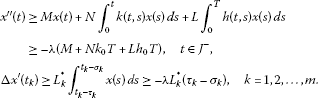If ${x}^{\prime }\left(t\right)>0$ for all $t\in J$, then $\mathrm{\Delta }x\left({t}_{k}\right)={L}_{k}{\int }_{{t}_{k}-{\delta }_{k}}^{{t}_{k}-{\epsilon }_{k}}{x}^{\prime }\left(s\right)\phantom{\rule{0.2em}{0ex}}ds\ge 0$, $k=1,2,\dots ,m$. Hence, $x\left(t\right)$ is strictly increasing on J, which contradicts $x\left(0\right)=x\left(T\right)$. Then there exists a $\overline{t}\in J$ such that ${x}^{\prime }\left(\overline{t}\right)\le 0$.

Let $\overline{t}\in {J}_{j}$, $j\in \left\{0,1,\dots ,m\right\}$. By mean value theorem, we have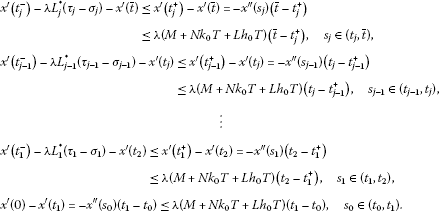Summing up the above inequalities, we obtain

$\begin{array}{rcl}{x}^{\prime }\left(0\right)& \le & {x}^{\prime }\left(\overline{t}\right)+\lambda \left[\sum _{k=1}^{j}{L}_{k}^{\ast }\left({\tau }_{k}-{\sigma }_{k}\right)+\left(M+N{k}_{0}T+L{h}_{0}T\right)\left(\overline{t}-{t}_{0}\right)\right]\\ \le & \lambda \left[\sum _{k=1}^{j}{L}_{k}^{\ast }\left({\tau }_{k}-{\sigma }_{k}\right)+\left(M+N{k}_{0}T+L{h}_{0}T\right)\overline{t}\right].\end{array}$
(2.3)

Let $t\in {J}_{h}$, $h\in \left\{0,1,\dots ,m\right\}$. If $t\le \overline{t}$ by using the method to get (2.3), then we have

$\begin{array}{rcl}{x}^{\prime }\left(t\right)& \le & {x}^{\prime }\left(\overline{t}\right)+\lambda \left[\sum _{k=h+1}^{j}{L}_{k}^{\ast }\left({\tau }_{k}-{\sigma }_{k}\right)+\left(M+N{k}_{0}T+L{h}_{0}T\right)\left(\overline{t}-t\right)\right]\\ \le & \lambda \left[\sum _{k=1}^{m}{L}_{k}^{\ast }\left({\tau }_{k}-{\sigma }_{k}\right)+\left(M+N{k}_{0}T+L{h}_{0}T\right)T\right].\end{array}$

If $t>\overline{t}$, then the above method together with (2.1), (2.3) implies that

$\begin{array}{rcl}{x}^{\prime }\left(t\right)& \le & {x}^{\prime }\left(T\right)+\lambda \left[\sum _{k=h+1}^{m}{L}_{k}^{\ast }\left({\tau }_{k}-{\sigma }_{k}\right)+\left(M+N{k}_{0}T+L{h}_{0}T\right)\left(T-t\right)\right]\\ \le & {x}^{\prime }\left(0\right)+\lambda \left[\sum _{k=h+1}^{m}{L}_{k}^{\ast }\left({\tau }_{k}-{\sigma }_{k}\right)+\left(M+N{k}_{0}T+L{h}_{0}T\right)\left(T-t\right)\right]\\ \le & \lambda \left[\sum _{k=1}^{j}{L}_{k}^{\ast }\left({\tau }_{k}-{\sigma }_{k}\right)+\left(M+N{k}_{0}T+L{h}_{0}T\right)\overline{t}\right]\\ +\lambda \left[\sum _{k=h+1}^{m}{L}_{k}^{\ast }\left({\tau }_{k}-{\sigma }_{k}\right)+\left(M+N{k}_{0}T+L{h}_{0}T\right)\left(T-t\right)\right]\\ \le & \lambda \left[\sum _{k=1}^{m}{L}_{k}^{\ast }\left({\tau }_{k}-{\sigma }_{k}\right)+\left(M+N{k}_{0}T+L{h}_{0}T\right)T\right].\end{array}$

Thus,

${x}^{\prime }\left(t\right)\le \lambda \left[\sum _{k=1}^{m}{L}_{k}^{\ast }\left({\tau }_{k}-{\sigma }_{k}\right)+\left(M+N{k}_{0}T+L{h}_{0}T\right)T\right],\phantom{\rule{1em}{0ex}}t\in {J}^{-}.$

Let ${t}^{\ast }\in {J}_{r}$ for some $r\in \left\{0,1,\dots ,m\right\}$. We first assume that ${t}_{\ast }<{t}^{\ast }$, then $i. By the mean value theorem, we have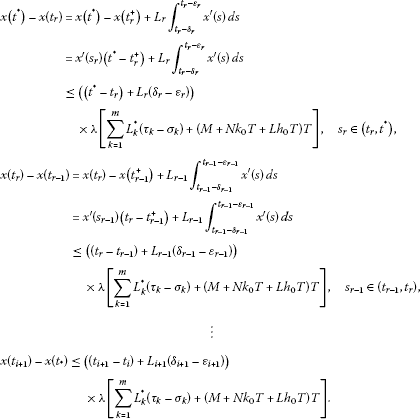Summing up, we get

$0

Hence,

$\left[\sum _{k=1}^{m}{L}_{k}\left({\delta }_{k}-{\epsilon }_{k}\right)+T\right]\left[\sum _{k=1}^{m}{L}_{k}^{\ast }\left({\tau }_{k}-{\sigma }_{k}\right)+\left(M+N{k}_{0}T+L{h}_{0}T\right)T\right]>1,$

For the case ${t}^{\ast }<{t}_{\ast }$, the proof is similar, and thus we omit it. This completes the proof. □

Lemma 2.2 Assume that $x\in E$ satisfies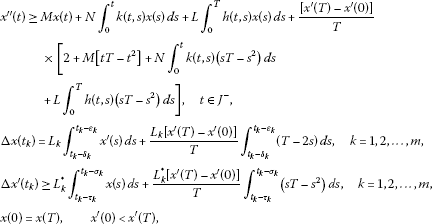where$M>0$, $N\ge 0$, $L\ge 0$, ${L}_{k}\ge 0$, ${L}_{k}^{\ast }\ge 0$are constants and$0\le {\epsilon }_{k}\le {\delta }_{k}\le {t}_{k}-{t}_{k-1}$, $0\le {\sigma }_{k}\le {\tau }_{k}\le {t}_{k}-{t}_{k-1}$, $k=1,2,\dots ,m$, and they satisfy (2.2). Then$x\left(t\right)\le 0$for all$t\in J$.

Proof Let $u\left(t\right)=\left[\frac{tT-{t}^{2}}{T}\right]\left[{x}^{\prime }\left(T\right)-{x}^{\prime }\left(0\right)\right]$, $t\in J$, and define

$w\left(t\right)=x\left(t\right)+u\left(t\right)=x\left(t\right)+\left[\frac{tT-{t}^{2}}{T}\right]\left[{x}^{\prime }\left(T\right)-{x}^{\prime }\left(0\right)\right].$

Note that $u\left(0\right)=u\left(T\right)$, $u\left(t\right)\ge 0$ for $t\in J$. If we prove that $w\le 0$, then $x\left(t\right)\le x\left(t\right)+u\left(t\right)\le 0$ and the proof is complete. Since ${u}^{\prime }\left(t\right)=\left[\frac{T-2t}{T}\right]\left[{x}^{\prime }\left(T\right)-{x}^{\prime }\left(0\right)\right]$, then we get

$\begin{array}{c}w\left(0\right)=x\left(0\right)+u\left(0\right)=x\left(T\right)+u\left(T\right)=w\left(T\right),\hfill \\ {w}^{\prime }\left(0\right)={x}^{\prime }\left(0\right)+{u}^{\prime }\left(0\right)={x}^{\prime }\left(0\right)+{x}^{\prime }\left(T\right)-{x}^{\prime }\left(0\right)={x}^{\prime }\left(T\right),\hfill \\ {w}^{\prime }\left(T\right)={x}^{\prime }\left(T\right)+{u}^{\prime }\left(T\right)={x}^{\prime }\left(T\right)-{x}^{\prime }\left(T\right)+{x}^{\prime }\left(0\right)={x}^{\prime }\left(0\right).\hfill \end{array}$

Hence, ${w}^{\prime }\left(0\right)>{w}^{\prime }\left(T\right)$. Indeed, for $k=1,2,\dots ,m$,

$\begin{array}{rcl}\mathrm{\Delta }w\left({t}_{k}\right)& =& \mathrm{\Delta }x\left({t}_{k}\right)+\mathrm{\Delta }u\left({t}_{k}\right)\\ =& {L}_{k}{\int }_{{t}_{k}-{\delta }_{k}}^{{t}_{k}-{\epsilon }_{k}}{x}^{\prime }\left(s\right)\phantom{\rule{0.2em}{0ex}}ds+\frac{{L}_{k}\left[{x}^{\prime }\left(T\right)-{x}^{\prime }\left(0\right)\right]}{T}{\int }_{{t}_{k}-{\delta }_{k}}^{{t}_{k}-{\epsilon }_{k}}T-2s\phantom{\rule{0.2em}{0ex}}ds\\ =& {L}_{k}{\int }_{{t}_{k}-{\delta }_{k}}^{{t}_{k}-{\epsilon }_{k}}{x}^{\prime }\left(s\right)+{u}^{\prime }\left(s\right)\phantom{\rule{0.2em}{0ex}}ds\\ =& {L}_{k}{\int }_{{t}_{k}-{\delta }_{k}}^{{t}_{k}-{\epsilon }_{k}}{w}^{\prime }\left(s\right)\phantom{\rule{0.2em}{0ex}}ds,\end{array}$

and

$\begin{array}{rcl}\mathrm{\Delta }{w}^{\prime }\left({t}_{k}\right)& =& \mathrm{\Delta }{x}^{\prime }\left({t}_{k}\right)+\mathrm{\Delta }{u}^{\prime }\left({t}_{k}\right)\\ \ge & {L}_{k}^{\ast }{\int }_{{t}_{k}-{\tau }_{k}}^{{t}_{k}-{\sigma }_{k}}x\left(s\right)\phantom{\rule{0.2em}{0ex}}ds+\frac{{L}_{k}^{\ast }\left[{x}^{\prime }\left(T\right)-{x}^{\prime }\left(0\right)\right]}{T}{\int }_{{t}_{k}-{\tau }_{k}}^{{t}_{k}-{\sigma }_{k}}sT-{s}^{2}\phantom{\rule{0.2em}{0ex}}ds\\ \ge & {L}_{k}^{\ast }{\int }_{{t}_{k}-{\tau }_{k}}^{{t}_{k}-{\sigma }_{k}}x\left(s\right)+u\left(s\right)\phantom{\rule{0.2em}{0ex}}ds\\ \ge & {L}_{k}^{\ast }{\int }_{{t}_{k}-{\tau }_{k}}^{{t}_{k}-{\sigma }_{k}}w\left(s\right)\phantom{\rule{0.2em}{0ex}}ds.\end{array}$

Meanwhile, for $t\ne {t}_{k}$, $t\in J$,

$\begin{array}{c}{w}^{″}\left(t\right)-Mw\left(t\right)-N{\int }_{0}^{t}k\left(t,s\right)w\left(s\right)\phantom{\rule{0.2em}{0ex}}ds-L{\int }_{0}^{T}h\left(t,s\right)w\left(s\right)\phantom{\rule{0.2em}{0ex}}ds\hfill \\ \phantom{\rule{1em}{0ex}}={x}^{″}\left(t\right)-Mx\left(t\right)-\frac{Mt}{T}\left[{x}^{\prime }\left(T\right)-{x}^{\prime }\left(0\right)\right]-N{\int }_{0}^{t}k\left(t,s\right)x\left(s\right)\phantom{\rule{0.2em}{0ex}}ds-L{\int }_{0}^{T}h\left(t,s\right)x\left(s\right)\phantom{\rule{0.2em}{0ex}}ds\hfill \\ \phantom{\rule{2em}{0ex}}-\frac{\left[{x}^{\prime }\left(T\right)-{x}^{\prime }\left(0\right)\right]}{T}\left[2+M\left[tT-{t}^{2}\right]+N{\int }_{0}^{t}k\left(t,s\right)\left(sT-{s}^{2}\right)\phantom{\rule{0.2em}{0ex}}ds\hfill \\ \phantom{\rule{2em}{0ex}}+L{\int }_{0}^{T}h\left(t,s\right)\left(sT-{s}^{2}\right)\phantom{\rule{0.2em}{0ex}}ds\right]\ge 0.\hfill \end{array}$

Then by Lemma 2.1, we get $w\left(t\right)\le 0$ for all $t\in J$, which implies that $x\left(t\right)\le 0$, $t\in J$. □

Consider the linear PBVP

$\left\{\begin{array}{c}{x}^{″}\left(t\right)=Mx\left(t\right)+N{\int }_{0}^{t}k\left(t,s\right)x\left(s\right)\phantom{\rule{0.2em}{0ex}}ds+L{\int }_{0}^{T}h\left(t,s\right)x\left(s\right)\phantom{\rule{0.2em}{0ex}}ds-g\left(t\right),\phantom{\rule{1em}{0ex}}t\in {J}^{-},\hfill \\ \mathrm{\Delta }x\left({t}_{k}\right)={L}_{k}{\int }_{{t}_{k}-{\delta }_{k}}^{{t}_{k}-{\epsilon }_{k}}{x}^{\prime }\left(s\right)\phantom{\rule{0.2em}{0ex}}ds+{\gamma }_{k},\phantom{\rule{1em}{0ex}}k=1,2,\dots ,m,\hfill \\ \mathrm{\Delta }{x}^{\prime }\left({t}_{k}\right)={L}_{k}^{\ast }{\int }_{{t}_{k}-{\tau }_{k}}^{{t}_{k}-{\sigma }_{k}}x\left(s\right)\phantom{\rule{0.2em}{0ex}}ds+{\lambda }_{k},\phantom{\rule{1em}{0ex}}k=1,2,\dots ,m,\hfill \\ x\left(0\right)=x\left(T\right),\phantom{\rule{2em}{0ex}}{x}^{\prime }\left(0\right)={x}^{\prime }\left(T\right),\hfill \end{array}$
(2.4)

where constants $M>0$, $N\ge 0$, $L\ge 0$, ${L}_{k}\ge 0$, ${L}_{k}^{\ast }\ge 0$, ${\gamma }_{k}$, ${\lambda }_{k}$ are constants and $g\in PC\left(J,R\right)$, $0\le {\epsilon }_{k}\le {\delta }_{k}\le {t}_{k}-{t}_{k-1}$, $0\le {\sigma }_{k}\le {\tau }_{k}\le {t}_{k}-{t}_{k-1}$, $k=1,2,\dots ,m$.

Lemma 2.3$x\in E$is a solution of (2.4) if and only if$x\in P{C}^{1}\left(J,R\right)$is a solution of the following impulsive integral equation:

$\begin{array}{rcl}x\left(t\right)& =& {\int }_{0}^{T}{G}_{1}\left(t,s\right)\left[g\left(s\right)-N{\int }_{0}^{s}k\left(s,r\right)x\left(r\right)\phantom{\rule{0.2em}{0ex}}dr-L{\int }_{0}^{T}h\left(s,r\right)x\left(r\right)\phantom{\rule{0.2em}{0ex}}dr\right]\phantom{\rule{0.2em}{0ex}}ds\\ +\sum _{k=1}^{m}\left[-{G}_{1}\left(t,{t}_{k}\right)\left({L}_{k}^{\ast }{\int }_{{t}_{k}-{\tau }_{k}}^{{t}_{k}-{\sigma }_{k}}x\left(s\right)\phantom{\rule{0.2em}{0ex}}ds+{\lambda }_{k}\right)\\ +{G}_{2}\left(t,{t}_{k}\right)\left({L}_{k}{\int }_{{t}_{k}-{\delta }_{k}}^{{t}_{k}-{\epsilon }_{k}}{x}^{\prime }\left(s\right)\phantom{\rule{0.2em}{0ex}}ds+{\gamma }_{k}\right)\right],\end{array}$
(2.5)

where

$\begin{array}{c}{G}_{1}\left(t,s\right)={\left[2\sqrt{M}\left({e}^{\sqrt{M}T}-1\right)\right]}^{-1}\left\{\begin{array}{cc}{e}^{\sqrt{M}\left(T-t+s\right)}+{e}^{\sqrt{M}\left(t-s\right)},\hfill & 0\le s

This proof is similar to the proof of Lemma 2.1 in , and we omit it.

Lemma 2.4 Let$M>0$, $N\ge 0$, $L\ge 0$, ${L}_{k}\ge 0$, ${L}_{k}^{\ast }\ge 0$are constants and$0\le {\epsilon }_{k}\le {\delta }_{k}\le {t}_{k}-{t}_{k-1}$, $0\le {\sigma }_{k}\le {\tau }_{k}\le {t}_{k}-{t}_{k-1}$, $k=1,2,\dots ,m$. If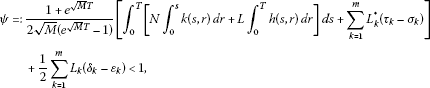(2.6)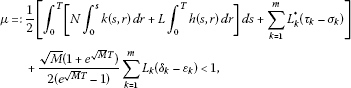(2.7)

then (2.4) has a unique solution x in E.

Proof For any $x\in E$, we define an operator F by

$\begin{array}{rcl}\left(Fx\right)\left(t\right)& =& {\int }_{0}^{T}{G}_{1}\left(t,s\right)\left[g\left(s\right)-N{\int }_{0}^{s}k\left(s,r\right)x\left(r\right)\phantom{\rule{0.2em}{0ex}}dr-L{\int }_{0}^{T}h\left(s,r\right)x\left(r\right)\phantom{\rule{0.2em}{0ex}}dr\right]\phantom{\rule{0.2em}{0ex}}ds\\ +\sum _{k=1}^{m}\left[-{G}_{1}\left(t,{t}_{k}\right)\left({L}_{k}^{\ast }{\int }_{{t}_{k}-{\tau }_{k}}^{{t}_{k}-{\sigma }_{k}}x\left(s\right)\phantom{\rule{0.2em}{0ex}}ds+{\lambda }_{k}\right)\\ +{G}_{2}\left(t,{t}_{k}\right)\left({L}_{k}{\int }_{{t}_{k}-{\delta }_{k}}^{{t}_{k}-{\epsilon }_{k}}{x}^{\prime }\left(s\right)\phantom{\rule{0.2em}{0ex}}ds+{\gamma }_{k}\right)\right],\end{array}$

where ${G}_{1}$, ${G}_{2}$ are given by Lemma 2.3. Then $Fx\in P{C}^{1}\left(J,R\right)$ and

$\begin{array}{rcl}{\left(Fx\right)}^{\prime }\left(t\right)& =& -{\int }_{0}^{T}{G}_{2}\left(t,s\right)\left[g\left(s\right)-N{\int }_{0}^{s}k\left(s,r\right)x\left(r\right)\phantom{\rule{0.2em}{0ex}}dr-L{\int }_{0}^{T}h\left(s,r\right)x\left(r\right)\phantom{\rule{0.2em}{0ex}}dr\right]\phantom{\rule{0.2em}{0ex}}ds\\ +\sum _{k=1}^{m}\left[{G}_{2}\left(t,{t}_{k}\right)\left({L}_{k}^{\ast }{\int }_{{t}_{k}-{\tau }_{k}}^{{t}_{k}-{\sigma }_{k}}x\left(s\right)\phantom{\rule{0.2em}{0ex}}ds+{\lambda }_{k}\right)\\ -M{G}_{1}\left(t,{t}_{k}\right)\left({L}_{k}{\int }_{{t}_{k}-{\delta }_{k}}^{{t}_{k}-{\epsilon }_{k}}{x}^{\prime }\left(s\right)\phantom{\rule{0.2em}{0ex}}ds+{\gamma }_{k}\right)\right].\end{array}$

By computing directly, we have

$\underset{\left(t,s\right)\in J×J}{max}\left\{{G}_{1}\left(t,s\right)\right\}=\frac{1+{e}^{\sqrt{M}T}}{2\sqrt{M}\left({e}^{\sqrt{M}T}-1\right)}$

and

$\underset{\left(t,s\right)\in J×J}{max}\left\{{G}_{2}\left(t,s\right)\right\}=\frac{1}{2}.$

On the other hand, for $x,y\in P{C}^{1}\left(J,R\right)$, we have

$\begin{array}{rcl}{\parallel \left(Fx\right)-\left(Fy\right)\parallel }_{PC}& =& \underset{t\in J}{sup}|\left(Fx\right)\left(t\right)-\left(Fy\right)\left(t\right)|\\ =& \underset{t\in J}{sup}|{\int }_{0}^{T}{G}_{1}\left(t,s\right)\left[-N{\int }_{0}^{s}k\left(s,r\right)\left[x\left(r\right)-y\left(r\right)\right]\phantom{\rule{0.2em}{0ex}}dr\\ -L{\int }_{0}^{T}h\left(s,r\right)\left[x\left(r\right)-y\left(r\right)\right]\phantom{\rule{0.2em}{0ex}}dr\right]\phantom{\rule{0.2em}{0ex}}ds\\ +\sum _{k=1}^{m}\left[-{G}_{1}\left(t,{t}_{k}\right)\left({L}_{k}^{\ast }{\int }_{{t}_{k}-{\tau }_{k}}^{{t}_{k}-{\sigma }_{k}}\left[x\left(s\right)-y\left(s\right)\right]\phantom{\rule{0.2em}{0ex}}ds\right)\\ +{G}_{2}\left(t,{t}_{k}\right)\left({L}_{k}{\int }_{{t}_{k}-{\delta }_{k}}^{{t}_{k}-{\epsilon }_{k}}\left[{x}^{\prime }\left(s\right)-{y}^{\prime }\left(s\right)\right]\phantom{\rule{0.2em}{0ex}}ds\right)\right]|\\ \le & \underset{t\in J}{sup}{\int }_{0}^{T}{G}_{1}\left(t,s\right)\left[N|x\left(s\right)-y\left(s\right)|{\int }_{0}^{s}k\left(s,r\right)\phantom{\rule{0.2em}{0ex}}dr\\ +L|x\left(s\right)-y\left(s\right)|{\int }_{0}^{T}h\left(s,r\right)\phantom{\rule{0.2em}{0ex}}dr\right]\phantom{\rule{0.2em}{0ex}}ds\\ +\sum _{k=1}^{m}\left[{G}_{1}\left(t,{t}_{k}\right){L}_{k}^{\ast }\left({\tau }_{k}-{\sigma }_{k}\right)|x\left(t\right)-y\left(t\right)|\\ +{G}_{2}\left(t,{t}_{k}\right){L}_{k}\left({\delta }_{k}-{\epsilon }_{k}\right)|{x}^{\prime }\left(t\right)-{y}^{\prime }\left(t\right)|\right]\\ \le & {\parallel x-y\parallel }_{P{C}^{1}}\left[\underset{t\in J}{sup}{\int }_{0}^{T}{G}_{1}\left(t,s\right)\left[N{\int }_{0}^{s}k\left(s,r\right)\phantom{\rule{0.2em}{0ex}}dr\\ +L{\int }_{0}^{T}h\left(s,r\right)\phantom{\rule{0.2em}{0ex}}dr\right]\phantom{\rule{0.2em}{0ex}}ds+\sum _{k=1}^{m}\left[{G}_{1}\left(t,{t}_{k}\right){L}_{k}^{\ast }\left({\tau }_{k}-{\sigma }_{k}\right)\\ +{G}_{2}\left(t,{t}_{k}\right){L}_{k}\left({\delta }_{k}-{\epsilon }_{k}\right)\right]\right]\\ \le & \psi {\parallel x-y\parallel }_{P{C}^{1}}.\end{array}$

Similarly,

$\begin{array}{rcl}{\parallel {\left(Fx\right)}^{\prime }-{\left(Fy\right)}^{\prime }\parallel }_{PC}& =& \underset{t\in J}{sup}|-{\int }_{0}^{T}{G}_{2}\left(t,s\right)\left[-N{\int }_{0}^{s}k\left(s,r\right)\left[x\left(r\right)-y\left(r\right)\right]\phantom{\rule{0.2em}{0ex}}dr\\ -L{\int }_{0}^{T}h\left(s,r\right)\left[x\left(r\right)-y\left(r\right)\right]\phantom{\rule{0.2em}{0ex}}dr\right]\phantom{\rule{0.2em}{0ex}}ds\\ +\sum _{k=1}^{m}\left[{G}_{2}\left(t,{t}_{k}\right)\left({L}_{k}^{\ast }{\int }_{{t}_{k}-{\tau }_{k}}^{{t}_{k}-{\sigma }_{k}}\left[x\left(s\right)-y\left(s\right)\right]\phantom{\rule{0.2em}{0ex}}ds\right)\\ -M{G}_{1}\left(t,{t}_{k}\right)\left({L}_{k}{\int }_{{t}_{k}-{\delta }_{k}}^{{t}_{k}-{\epsilon }_{k}}\left[{x}^{\prime }\left(s\right)-{y}^{\prime }\left(s\right)\right]\phantom{\rule{0.2em}{0ex}}ds\right)\right]|\\ \le & \underset{t\in J}{sup}{\int }_{0}^{T}{G}_{2}\left(t,s\right)\left[N|x\left(s\right)-y\left(s\right)|{\int }_{0}^{s}k\left(s,r\right)\phantom{\rule{0.2em}{0ex}}dr\\ +L|x\left(s\right)-y\left(s\right)|{\int }_{0}^{T}h\left(s,r\right)\phantom{\rule{0.2em}{0ex}}dr\right]\phantom{\rule{0.2em}{0ex}}ds\\ +\sum _{k=1}^{m}\left[{G}_{2}\left(t,{t}_{k}\right){L}_{k}^{\ast }\left({\tau }_{k}-{\sigma }_{k}\right)|x\left(t\right)-y\left(t\right)|\\ +M{G}_{1}\left(t,{t}_{k}\right){L}_{k}\left({\delta }_{k}-{\epsilon }_{k}\right)|{x}^{\prime }\left(s\right)-{y}^{\prime }\left(s\right)|\right]\\ \le & {\parallel x-y\parallel }_{P{C}^{1}}\left[\underset{t\in J}{sup}{\int }_{0}^{T}{G}_{2}\left(t,s\right)\left[N{\int }_{0}^{s}k\left(s,r\right)\phantom{\rule{0.2em}{0ex}}dr\\ +L{\int }_{0}^{T}h\left(s,r\right)\phantom{\rule{0.2em}{0ex}}dr\right]\phantom{\rule{0.2em}{0ex}}ds+\sum _{k=1}^{m}\left[{G}_{2}\left(t,{t}_{k}\right){L}_{k}^{\ast }\left({\tau }_{k}-{\sigma }_{k}\right)\\ +M{G}_{1}\left(t,{t}_{k}\right){L}_{k}\left({\delta }_{k}-{\epsilon }_{k}\right)\right]\right]\\ \le & \mu {\parallel x-y\parallel }_{P{C}^{1}}.\end{array}$

Thus,

${\parallel \left(Fx\right)-\left(Fy\right)\parallel }_{P{C}^{1}}\le max\left\{\psi ,\mu \right\}{\parallel x-y\parallel }_{P{C}^{1}}.$

By the Banach fixed-point theorem, F has a unique fixed point ${x}^{\ast }\in P{C}^{1}\left(J,R\right)$, and by Lemma 2.3, ${x}^{\ast }$ is also the unique solution of (2.4). This completes the proof. □

## 3 Main results

In this section, we establish existence criteria for solutions of PBVP (1.1) by the method of lower and upper solutions and the monotone iterative technique. For ${\alpha }_{0},{\beta }_{0}\in E$, we write ${\alpha }_{0}\le {\beta }_{0}$ if ${\alpha }_{0}\left(t\right)\le {\beta }_{0}\left(t\right)$ for all $t\in J$. In such a case, we denote $\left[{\alpha }_{0},{\beta }_{0}\right]=\left\{x\in E:{\alpha }_{0}\left(t\right)\le x\left(t\right)\le {\beta }_{0}\left(t\right),t\in J\right\}$.

Theorem 3.1 Suppose that the following conditions hold:

(H1) ${\alpha }_{0}$and${\beta }_{0}$are lower and upper solutions for PBVP (1.1), respectively, such that${\alpha }_{0}\le {\beta }_{0}$.

(H2) The function f satisfies

$f\left(t,{x}_{2},{y}_{2},{z}_{2}\right)-f\left(t,{x}_{1},{y}_{1},{z}_{1}\right)\le M\left({x}_{2}-{x}_{1}\right)+N\left({y}_{2}-{y}_{1}\right)+L\left({z}_{2}-{z}_{1}\right),$

for all$t\in J$, ${\alpha }_{0}\left(t\right)\le {x}_{1}\le {x}_{2}\le {\beta }_{0}\left(t\right)$, $\left(K{\alpha }_{0}\right)\left(t\right)\le {y}_{1}\le {y}_{2}\le \left(K{\beta }_{0}\right)\left(t\right)$, $\left(S{\alpha }_{0}\right)\left(t\right)\le {z}_{1}\le {z}_{2}\le \left(S{\beta }_{0}\right)\left(t\right)$.

(H3) $M>0$, $N\ge 0$, $L\ge 0$, ${L}_{k}\ge 0$, ${L}_{k}^{\ast }\ge 0$are constants, and$0\le {\epsilon }_{k}\le {\delta }_{k}\le {t}_{k}-{t}_{k-1}$, $0\le {\sigma }_{k}\le {\tau }_{k}\le {t}_{k}-{t}_{k-1}$, $k=1,2,\dots ,m$, and they satisfy (2.2), (2.6) and (2.7).

(H4) The functions${I}_{k}$, ${I}_{k}^{\ast }$satisfy

$\begin{array}{c}{I}_{k}\left({\int }_{{t}_{k}-{\delta }_{k}}^{{t}_{k}-{\epsilon }_{k}}{x}^{\prime }\left(s\right)\phantom{\rule{0.2em}{0ex}}ds\right)-{I}_{k}\left({\int }_{{t}_{k}-{\delta }_{k}}^{{t}_{k}-{\epsilon }_{k}}{y}^{\prime }\left(s\right)\phantom{\rule{0.2em}{0ex}}ds\right)={L}_{k}{\int }_{{t}_{k}-{\delta }_{k}}^{{t}_{k}-{\epsilon }_{k}}{x}^{\prime }\left(s\right)-{y}^{\prime }\left(s\right)\phantom{\rule{0.2em}{0ex}}ds,\hfill \\ {I}_{k}^{\ast }\left({\int }_{{t}_{k}-{\tau }_{k}}^{{t}_{k}-{\sigma }_{k}}x\left(s\right)\phantom{\rule{0.2em}{0ex}}ds\right)-{I}_{k}^{\ast }\left({\int }_{{t}_{k}-{\tau }_{k}}^{{t}_{k}-{\sigma }_{k}}y\left(s\right)\phantom{\rule{0.2em}{0ex}}ds\right)\le {L}_{k}^{\ast }{\int }_{{t}_{k}-{\tau }_{k}}^{{t}_{k}-{\sigma }_{k}}x\left(s\right)-y\left(s\right)\phantom{\rule{0.2em}{0ex}}ds,\hfill \end{array}$

where${\int }_{{t}_{k}-{\tau }_{k}}^{{t}_{k}-{\sigma }_{k}}{\alpha }_{0}\left(s\right)\phantom{\rule{0.2em}{0ex}}ds\le {\int }_{{t}_{k}-{\tau }_{k}}^{{t}_{k}-{\sigma }_{k}}y\left(s\right)\phantom{\rule{0.2em}{0ex}}ds\le {\int }_{{t}_{k}-{\tau }_{k}}^{{t}_{k}-{\sigma }_{k}}x\left(s\right)\phantom{\rule{0.2em}{0ex}}ds\le {\int }_{{t}_{k}-{\tau }_{k}}^{{t}_{k}-{\sigma }_{k}}{\beta }_{0}\left(s\right)\phantom{\rule{0.2em}{0ex}}ds$, $0\le {\sigma }_{k}\le {\tau }_{k}\le {t}_{k}-{t}_{k-1}$, $0\le {\epsilon }_{k}\le {\delta }_{k}\le {t}_{k}-{t}_{k-1}$, $k=1,2,\dots ,m$.

Then there exist monotone sequences$\left\{{\alpha }_{n}\right\},\left\{{\beta }_{n}\right\}\subset E$which converge in E to the extreme solutions of PBVP (1.1) in$\left[{\alpha }_{0},{\beta }_{0}\right]$, respectively.

Proof For any $\eta \in \left[{\alpha }_{0},{\beta }_{0}\right]$, we consider linear PBVP (2.4) with

$\begin{array}{c}g\left(t\right)=f\left(t,\eta \left(t\right),\left(K\eta \right)\left(t\right),\left(S\eta \right)\left(t\right)\right)-M\eta \left(t\right)-N{\int }_{0}^{t}k\left(t,s\right)\eta \left(s\right)\phantom{\rule{0.2em}{0ex}}ds-L{\int }_{0}^{T}h\left(t,s\right)\eta \left(s\right)\phantom{\rule{0.2em}{0ex}}ds,\hfill \\ {\gamma }_{k}={I}_{k}\left({\int }_{{t}_{k}-{\delta }_{k}}^{{t}_{k}-{\epsilon }_{k}}{\eta }^{\prime }\left(s\right)\phantom{\rule{0.2em}{0ex}}ds\right)-{L}_{k}{\int }_{{t}_{k}-{\delta }_{k}}^{{t}_{k}-{\epsilon }_{k}}{\eta }^{\prime }\left(s\right)\phantom{\rule{0.2em}{0ex}}ds,\phantom{\rule{1em}{0ex}}k=1,2,\dots ,m,\hfill \\ {\lambda }_{k}={I}_{k}^{\ast }\left({\int }_{{t}_{k}-{\tau }_{k}}^{{t}_{k}-{\sigma }_{k}}\eta \left(s\right)\phantom{\rule{0.2em}{0ex}}ds\right)-{L}_{k}^{\ast }{\int }_{{t}_{k}-{\tau }_{k}}^{{t}_{k}-{\sigma }_{k}}\eta \left(s\right)\phantom{\rule{0.2em}{0ex}}ds,\phantom{\rule{1em}{0ex}}k=1,2,\dots ,m.\hfill \end{array}$

By Lemma 2.4, PBVP (2.4) has a unique solution $x\in E$. We define an operator A from $\left[{\alpha }_{0},{\beta }_{0}\right]$ to E by $x\left(t\right)=A\eta \left(t\right)$. We complete the proof in four steps.

Step 1. We claim that ${\alpha }_{0}\le A{\alpha }_{0}$ and $A{\beta }_{0}\le {\beta }_{0}$. We only prove ${\alpha }_{0}\le A{\alpha }_{0}$ since the second inequality can be proved in a similar manner.

Let ${\alpha }_{1}=A{\alpha }_{0}$ and $p={\alpha }_{0}-{\alpha }_{1}$. Then ${\alpha }_{1}$ satisfies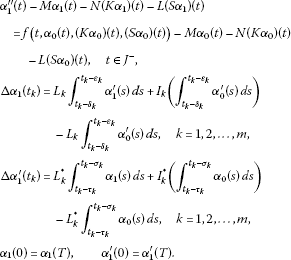We finish Step 1 in two cases.

Case 1. ${\alpha }_{0}^{\prime }\left(0\right)\ge {\alpha }_{0}^{\prime }\left(T\right)$, which implies that

$a\left(t\right)=0,\phantom{\rule{2em}{0ex}}{\alpha }_{0}^{″}\left(t\right)\ge f\left(t,{\alpha }_{0}\left(t\right),\left(K{\alpha }_{0}\right)\left(t\right),\left(S{\alpha }_{0}\right)\left(t\right)\right).$

As ${\alpha }_{0}$ is a lower solution of PBVP (1.1), then for $t\in {J}^{-}$,

$\begin{array}{c}{p}^{″}\left(t\right)-Mp\left(t\right)-N\left(Kp\right)\left(t\right)-L\left(Sp\right)\left(t\right)\hfill \\ \phantom{\rule{1em}{0ex}}={\alpha }_{0}^{″}\left(t\right)-M{\alpha }_{0}\left(t\right)-N\left(K{\alpha }_{0}\right)\left(t\right)-L\left(S{\alpha }_{0}\right)\left(t\right)\hfill \\ \phantom{\rule{2em}{0ex}}-{\alpha }_{1}^{″}\left(t\right)+M{\alpha }_{1}\left(t\right)+N\left(K{\alpha }_{1}\right)\left(t\right)+L\left(S{\alpha }_{1}\right)\left(t\right)\hfill \\ \phantom{\rule{1em}{0ex}}\ge f\left(t,{\alpha }_{0}\left(t\right),\left(K{\alpha }_{0}\right)\left(t\right),\left(S{\alpha }_{0}\right)\left(t\right)\right)-M{\alpha }_{0}\left(t\right)-N\left(K{\alpha }_{0}\right)\left(t\right)\hfill \\ \phantom{\rule{2em}{0ex}}-L\left(S{\alpha }_{0}\right)\left(t\right)-f\left(t,{\alpha }_{0}\left(t\right),\left(K{\alpha }_{0}\right)\left(t\right),\left(S{\alpha }_{0}\right)\left(t\right)\right)+M{\alpha }_{0}\left(t\right)\hfill \\ \phantom{\rule{2em}{0ex}}+N\left(K{\alpha }_{0}\right)\left(t\right)+L\left(S{\alpha }_{0}\right)\left(t\right)\hfill \\ \phantom{\rule{1em}{0ex}}=0,\hfill \end{array}$

and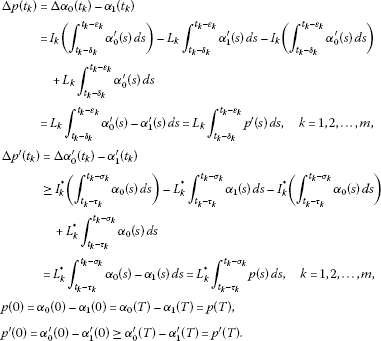Then by Lemma 2.1, $p\left(t\right)\le 0$, which implies that ${\alpha }_{0}\left(t\right)\le A{\alpha }_{0}\left(t\right)$, i.e., ${\alpha }_{0}\le A{\alpha }_{0}$.

Case 2. ${\alpha }_{0}^{\prime }\left(0\right)<{\alpha }_{0}^{\prime }\left(T\right)$, which implies that

$\begin{array}{rcl}a\left(t\right)& =& \frac{\left[{\alpha }_{0}^{\prime }\left(T\right)-{\alpha }_{0}^{\prime }\left(0\right)\right]}{T}\left[2+M\left[tT-{t}^{2}\right]+N{\int }_{0}^{t}k\left(t,s\right)\left(sT-{s}^{2}\right)\phantom{\rule{0.2em}{0ex}}ds\\ +L{\int }_{0}^{T}h\left(t,s\right)\left(sT-{s}^{2}\right)\phantom{\rule{0.2em}{0ex}}ds\right].\end{array}$

Hence,

$\begin{array}{c}{p}^{″}\left(t\right)-Mp\left(t\right)-N\left(Kp\right)\left(t\right)-L\left(Sp\right)\left(t\right)-\frac{\left[{p}^{\prime }\left(T\right)-{p}^{\prime }\left(0\right)\right]}{T}\left[2+M\left[tT-{t}^{2}\right]\hfill \\ \phantom{\rule{2em}{0ex}}+N{\int }_{0}^{t}k\left(t,s\right)\left(sT-{s}^{2}\right)\phantom{\rule{0.2em}{0ex}}ds+L{\int }_{0}^{T}h\left(t,s\right)\left(sT-{s}^{2}\right)\phantom{\rule{0.2em}{0ex}}ds\right]\hfill \\ \phantom{\rule{1em}{0ex}}={\alpha }_{0}^{″}\left(t\right)-M{\alpha }_{0}\left(t\right)-N\left(K{\alpha }_{0}\right)\left(t\right)-L\left(S{\alpha }_{0}\right)\left(t\right)-\frac{\left[{\alpha }_{0}^{\prime }\left(T\right)-{\alpha }_{0}^{\prime }\left(0\right)\right]}{T}\left[2+M\left[tT-{t}^{2}\right]\hfill \\ \phantom{\rule{2em}{0ex}}+N{\int }_{0}^{t}k\left(t,s\right)\left(sT-{s}^{2}\right)\phantom{\rule{0.2em}{0ex}}ds+L{\int }_{0}^{T}h\left(t,s\right)\left(sT-{s}^{2}\right)\phantom{\rule{0.2em}{0ex}}ds\right]\hfill \\ \phantom{\rule{2em}{0ex}}-{\alpha }_{1}^{″}\left(t\right)+M{\alpha }_{1}\left(t\right)+N\left(K{\alpha }_{1}\right)\left(t\right)+L\left(S{\alpha }_{1}\right)\left(t\right)\hfill \\ \phantom{\rule{1em}{0ex}}\ge f\left(t,{\alpha }_{0}\left(t\right),\left(K{\alpha }_{0}\right)\left(t\right),\left(S{\alpha }_{0}\right)\left(t\right)\right)-M{\alpha }_{0}\left(t\right)-N\left(K{\alpha }_{0}\right)\left(t\right)\hfill \\ \phantom{\rule{2em}{0ex}}-L\left(S{\alpha }_{0}\right)\left(t\right)-f\left(t,{\alpha }_{0}\left(t\right),\left(K{\alpha }_{0}\right)\left(t\right),\left(S{\alpha }_{0}\right)\left(t\right)\right)\hfill \\ \phantom{\rule{2em}{0ex}}+M{\alpha }_{0}\left(t\right)+N\left(K{\alpha }_{0}\right)\left(t\right)+L\left(S{\alpha }_{0}\right)\left(t\right)=0,\hfill \end{array}$

and

$\begin{array}{rcl}\mathrm{\Delta }p\left({t}_{k}\right)& =& \mathrm{\Delta }{\alpha }_{0}\left({t}_{k}\right)-\mathrm{\Delta }{\alpha }_{1}\left({t}_{k}\right)\\ =& {I}_{k}\left({\int }_{{t}_{k}-{\delta }_{k}}^{{t}_{k}-{\epsilon }_{k}}{\alpha }_{0}^{\prime }\left(s\right)\phantom{\rule{0.2em}{0ex}}ds\right)+\frac{{L}_{k}\left[{\alpha }_{0}^{\prime }\left(T\right)-{\alpha }_{0}^{\prime }\left(0\right)\right]}{T}{\int }_{{t}_{k}-{\delta }_{k}}^{{t}_{k}-{\epsilon }_{k}}T-2s\phantom{\rule{0.2em}{0ex}}ds\\ -{L}_{k}{\int }_{{t}_{k}-{\delta }_{k}}^{{t}_{k}-{\epsilon }_{k}}{\alpha }_{1}^{\prime }\left(s\right)\phantom{\rule{0.2em}{0ex}}ds-{I}_{k}\left({\int }_{{t}_{k}-{\delta }_{k}}^{{t}_{k}-{\epsilon }_{k}}{\alpha }_{0}^{\prime }\left(s\right)\phantom{\rule{0.2em}{0ex}}ds\right)+{L}_{k}{\int }_{{t}_{k}-{\delta }_{k}}^{{t}_{k}-{\epsilon }_{k}}{\alpha }_{0}^{\prime }\left(s\right)\phantom{\rule{0.2em}{0ex}}ds\\ =& {L}_{k}{\int }_{{t}_{k}-{\delta }_{k}}^{{t}_{k}-{\epsilon }_{k}}{p}^{\prime }\left(s\right)\phantom{\rule{0.2em}{0ex}}ds+\frac{{L}_{k}\left[{p}^{\prime }\left(T\right)-{p}^{\prime }\left(0\right)\right]}{T}{\int }_{{t}_{k}-{\delta }_{k}}^{{t}_{k}-{\epsilon }_{k}}T-2s\phantom{\rule{0.2em}{0ex}}ds,\phantom{\rule{1em}{0ex}}k=1,2,\dots ,m,\end{array}$

and

$\begin{array}{rcl}\mathrm{\Delta }{p}^{\prime }\left({t}_{k}\right)& =& \mathrm{\Delta }{\alpha }_{0}^{\prime }\left({t}_{k}\right)-{\alpha }_{1}^{\prime }\left({t}_{k}\right)\\ \ge & {I}_{k}^{\ast }\left({\int }_{{t}_{k}-{\tau }_{k}}^{{t}_{k}-{\sigma }_{k}}{\alpha }_{0}\left(s\right)\phantom{\rule{0.2em}{0ex}}ds\right)+\frac{{L}_{k}^{\ast }\left[{\alpha }_{0}^{\prime }\left(T\right)-{\alpha }_{0}^{\prime }\left(0\right)\right]}{T}{\int }_{{t}_{k}-{\tau }_{k}}^{{t}_{k}-{\sigma }_{k}}sT-{s}^{2}\phantom{\rule{0.2em}{0ex}}ds\\ -{L}_{k}^{\ast }{\int }_{{t}_{k}-{\tau }_{k}}^{{t}_{k}-{\sigma }_{k}}{\alpha }_{1}\left(s\right)\phantom{\rule{0.2em}{0ex}}ds-{I}_{k}^{\ast }\left({\int }_{{t}_{k}-{\tau }_{k}}^{{t}_{k}-{\sigma }_{k}}{\alpha }_{0}\left(s\right)\phantom{\rule{0.2em}{0ex}}ds\right)+{L}_{k}^{\ast }{\int }_{{t}_{k}-{\tau }_{k}}^{{t}_{k}-{\sigma }_{k}}{\alpha }_{0}\left(s\right)\phantom{\rule{0.2em}{0ex}}ds\\ =& {L}_{k}^{\ast }{\int }_{{t}_{k}-{\tau }_{k}}^{{t}_{k}-{\sigma }_{k}}p\left(s\right)\phantom{\rule{0.2em}{0ex}}ds+\frac{{L}_{k}^{\ast }\left[{p}^{\prime }\left(T\right)-{p}^{\prime }\left(0\right)\right]}{T}{\int }_{{t}_{k}-{\tau }_{k}}^{{t}_{k}-{\sigma }_{k}}sT-{s}^{2}\phantom{\rule{0.2em}{0ex}}ds,\end{array}$

$k=1,2,\dots ,m$, and

$p\left(0\right)=p\left(T\right),\phantom{\rule{2em}{0ex}}{p}^{\prime }\left(0\right)={\alpha }_{0}^{\prime }\left(0\right)-{\alpha }_{1}^{\prime }\left(0\right)<{\alpha }_{0}^{\prime }\left(T\right)-{\alpha }_{1}^{\prime }\left(T\right)={p}^{\prime }\left(T\right).$

Then by Lemma 2.2, $p\left(t\right)\le 0$, which implies ${\alpha }_{0}\left(t\right)\le A{\alpha }_{0}\left(t\right)$, i.e., ${\alpha }_{0}\le A{\alpha }_{0}$.

Step 2. We prove that if ${\alpha }_{0}\le {\eta }_{1}\le {\eta }_{2}\le {\beta }_{0}$, then $A{\eta }_{1}\le A{\eta }_{2}$.

Let ${\eta }_{1}^{\ast }=A{\eta }_{1}$, ${\eta }_{2}^{\ast }=A{\eta }_{2}$, and $p={\eta }_{1}^{\ast }-{\eta }_{2}^{\ast }$, then for $t\in {J}^{-}$, and by (H2), we obtain

$\begin{array}{c}{p}^{″}\left(t\right)-Mp\left(t\right)-N\left(Kp\right)\left(t\right)-L\left(Sp\right)\left(t\right)\hfill \\ \phantom{\rule{1em}{0ex}}=f\left(t,{\eta }_{1}\left(t\right),\left(K{\eta }_{1}\right)\left(t\right),\left(S{\eta }_{1}\right)\left(t\right)\right)-M{\eta }_{1}\left(t\right)-N\left(K{\eta }_{1}\right)\left(t\right)\hfill \\ \phantom{\rule{2em}{0ex}}-L\left(S{\eta }_{1}\right)\left(t\right)-f\left(t,{\eta }_{2}\left(t\right),\left(K{\eta }_{2}\right)\left(t\right),\left(S{\eta }_{2}\right)\left(t\right)\right)\hfill \\ \phantom{\rule{2em}{0ex}}+M{\eta }_{2}\left(t\right)+N\left(K{\eta }_{2}\right)\left(t\right)+L\left(S{\eta }_{2}\right)\left(t\right)\hfill \\ \phantom{\rule{1em}{0ex}}\ge 0\phantom{\rule{1em}{0ex}}\left(\text{by (H}{}_{2}\text{)}\right).\hfill \end{array}$

From (H3), we obtain

$\begin{array}{c}\mathrm{\Delta }p\left({t}_{k}\right)={L}_{k}{\int }_{{t}_{k}-{\delta }_{k}}^{{t}_{k}-{\epsilon }_{k}}{p}^{\prime }\left(s\right)\phantom{\rule{0.2em}{0ex}}ds,\phantom{\rule{1em}{0ex}}k=1,2,\dots ,m,\hfill \\ \mathrm{\Delta }{p}^{\prime }\left({t}_{k}\right)\ge {L}_{k}^{\ast }{\int }_{{t}_{k}-{\tau }_{k}}^{{t}_{k}-{\sigma }_{k}}p\left(s\right)\phantom{\rule{0.2em}{0ex}}ds,\phantom{\rule{1em}{0ex}}k=1,2,\dots ,m,\hfill \\ p\left(0\right)=p\left(T\right),\phantom{\rule{2em}{0ex}}{p}^{\prime }\left(0\right)={p}^{\prime }\left(T\right).\hfill \end{array}$

Applying Lemma 2.1, we get $p\left(t\right)\le 0$, which implies $A{\eta }_{1}\le A{\eta }_{2}$.

Step 3. We show that PBVP (1.1) has solutions.

Let ${\alpha }_{n}=A{\alpha }_{n-1}$, ${\beta }_{n}=A{\beta }_{n-1}$, $n=1,2,\dots$ . Following the first two steps, we have

${\alpha }_{0}\le {\alpha }_{1}\le \cdots \le {\alpha }_{n}\le \cdots \le {\beta }_{n}\le \cdots \le {\beta }_{1}\le {\beta }_{0},\phantom{\rule{1em}{0ex}}\mathrm{\forall }n\in N.$

Obviously, each ${\alpha }_{i}$, ${\beta }_{i}$ ($i=1,2,\dots$) satisfies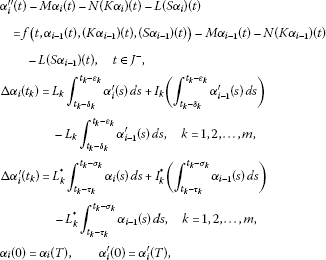and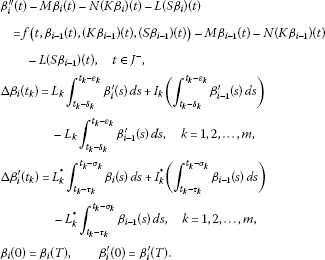Thus, there exist ${x}_{\ast }$ and ${x}^{\ast }$ such that

Clearly, ${x}_{\ast }$, ${x}^{\ast }$ satisfy PBVP (1.1).

Step 4. We show that ${x}_{\ast }$, ${x}^{\ast }$ are extreme solutions of PBVP (1.1).

Let $x\left(t\right)$ be any solution of PBVP (1.1), which satisfies ${\alpha }_{0}\left(t\right)\le x\left(t\right)\le {\beta }_{0}\left(t\right)$, $t\in J$. Suppose that there exists a positive integer n such that for $t\in J$, ${\alpha }_{n}\left(t\right)\le x\left(t\right)\le {\beta }_{n}\left(t\right)$. Setting $p\left(t\right)={\alpha }_{n+1}\left(t\right)-x\left(t\right)$, then for $t\in {J}^{-}$,

$\begin{array}{rcl}{p}^{″}\left(t\right)& =& {\alpha }_{n+1}^{″}-{x}^{″}\left(t\right)\\ =& M{\alpha }_{n+1}\left(t\right)+N\left(K{\alpha }_{n+1}\right)\left(t\right)+L\left(S{\alpha }_{n+1}\right)\left(t\right)\\ +f\left(t,{\alpha }_{n}\left(t\right),\left(K{\alpha }_{n}\right)\left(t\right),\left(S{\alpha }_{n}\right)\left(t\right)\right)-M{\alpha }_{n}\left(t\right)\\ -N\left(K{\alpha }_{n}\right)\left(t\right)-L\left(S{\alpha }_{n}\right)\left(t\right)\\ -f\left(t,x\left(t\right),\left(Kx\right)\left(t\right),\left(Sx\right)\left(t\right)\right)\\ =& M{\alpha }_{n+1}\left(t\right)+N\left(K{\alpha }_{n+1}\right)\left(t\right)+L\left(S{\alpha }_{n+1}\right)\left(t\right)-Mx\left(t\right)\\ -N\left(Kx\right)\left(t\right)-L\left(Sx\right)\left(t\right)+f\left(t,{\alpha }_{n}\left(t\right),\left(K{\alpha }_{n}\right)\left(t\right),\left(S{\alpha }_{n}\right)\left(t\right)\right)\\ -f\left(t,x\left(t\right),\left(Kx\right)\left(t\right),\left(Sx\right)\left(t\right)\right)-M\left({\alpha }_{n}\left(t\right)-x\left(t\right)\right)\\ -N\left(K\left({\alpha }_{n}\right)\left(t\right)-\left(Kx\right)\left(t\right)\right)-L\left(\left(S{\alpha }_{n}\right)\left(t\right)-\left(Sx\right)\left(t\right)\right)\\ \ge & Mp\left(t\right)+N\left(Kp\right)\left(t\right)+L\left(Sp\right)\left(t\right),\end{array}$

and

$\mathrm{\Delta }p\left({t}_{k}\right)=\mathrm{\Delta }{\alpha }_{n+1}\left({t}_{k}\right)-\mathrm{\Delta }x\left({t}_{k}\right)={L}_{k}{\int }_{{t}_{k}-{\delta }_{k}}^{{t}_{k}-{\epsilon }_{k}}{p}^{\prime }\left(s\right)\phantom{\rule{0.2em}{0ex}}ds,\phantom{\rule{1em}{0ex}}k=1,2,\dots ,m,$

and

$\begin{array}{rcl}\mathrm{\Delta }{p}^{\prime }\left({t}_{k}\right)& =& \mathrm{\Delta }{\alpha }_{n+1}^{\prime }\left({t}_{k}\right)-\mathrm{\Delta }{x}^{\prime }\left({t}_{k}\right)\\ =& {L}_{k}^{\ast }{\int }_{{t}_{k}-{\tau }_{k}}^{{t}_{k}-{\sigma }_{k}}{\alpha }_{n+1}\left(s\right)\phantom{\rule{0.2em}{0ex}}ds+{I}_{k}^{\ast }\left({\int }_{{t}_{k}-{\tau }_{k}}^{{t}_{k}-{\sigma }_{k}}{\alpha }_{n}\left(s\right)\phantom{\rule{0.2em}{0ex}}ds\right)\\ -{L}_{k}^{\ast }{\int }_{{t}_{k}-{\tau }_{k}}^{{t}_{k}-{\sigma }_{k}}{\alpha }_{n}\left(s\right)\phantom{\rule{0.2em}{0ex}}ds-{I}_{k}^{\ast }\left({\int }_{{t}_{k}-{\tau }_{k}}^{{t}_{k}-{\sigma }_{k}}x\left(s\right)\phantom{\rule{0.2em}{0ex}}ds\right)\\ \ge & {L}_{k}^{\ast }{\int }_{{t}_{k}-{\tau }_{k}}^{{t}_{k}-{\sigma }_{k}}{\alpha }_{n+1}\left(s\right)\phantom{\rule{0.2em}{0ex}}ds+{L}_{k}^{\ast }{\int }_{{t}_{k}-{\tau }_{k}}^{{t}_{k}-{\sigma }_{k}}{\alpha }_{n}\left(s\right)\phantom{\rule{0.2em}{0ex}}ds\\ -{L}_{k}^{\ast }{\int }_{{t}_{k}-{\tau }_{k}}^{{t}_{k}-{\sigma }_{k}}{\alpha }_{n}\left(s\right)\phantom{\rule{0.2em}{0ex}}ds-{L}_{k}^{\ast }{\int }_{{t}_{k}-{\tau }_{k}}^{{t}_{k}-{\sigma }_{k}}x\left(s\right)\phantom{\rule{0.2em}{0ex}}ds\\ =& {L}_{k}^{\ast }{\int }_{{t}_{k}-{\tau }_{k}}^{{t}_{k}-{\sigma }_{k}}p\left(s\right)\phantom{\rule{0.2em}{0ex}}ds,\phantom{\rule{1em}{0ex}}k=1,2,\dots ,m,\end{array}$

and

$p\left(0\right)=p\left(T\right),\phantom{\rule{2em}{0ex}}{p}^{\prime }\left(0\right)={p}^{\prime }\left(T\right).$

Still by Lemma 2.1, we have for all $t\in J$, $p\left(t\right)\le 0$, i.e., ${\alpha }_{n+1}\left(t\right)\le x\left(t\right)$. Similarly, we can prove that $x\left(t\right)\le {\beta }_{n+1}\left(t\right)$, $t\in J$. Therefore, ${\alpha }_{n+1}\left(t\right)\le x\left(t\right)\le {\beta }_{n+1}\left(t\right)$, for all $t\in J$, which implies ${x}_{\ast }\left(t\right)\le x\left(t\right)\le {x}^{\ast }\left(t\right)$. The proof is complete. □

## 4 An example

In this section, in order to illustrate our results, we consider an example.

Example 4.1 Consider the following PBVP:

$\left\{\begin{array}{c}{u}^{″}\left(t\right)=\frac{1}{4}{t}^{3}\left(u\left(t\right)-2\right)+\frac{5}{18}{\left[{\int }_{0}^{t}{t}^{2}{s}^{4}u\left(s\right)\phantom{\rule{0.2em}{0ex}}ds\right]}^{2}\hfill \\ \phantom{{u}^{″}\left(t\right)=}+\frac{1}{8}{\left[{\int }_{0}^{1}{t}^{3}{s}^{2}u\left(s\right)\phantom{\rule{0.2em}{0ex}}ds\right]}^{2},\phantom{\rule{1em}{0ex}}t\in J=\left[0,1\right],t\ne \frac{1}{2},\hfill \\ \mathrm{\Delta }u\left(\frac{1}{2}\right)=\frac{1}{2}{\int }_{\frac{1}{6}}^{\frac{3}{10}}{u}^{\prime }\left(s\right)\phantom{\rule{0.2em}{0ex}}ds,\phantom{\rule{1em}{0ex}}k=1,\hfill \\ \mathrm{\Delta }{u}^{\prime }\left(\frac{1}{2}\right)=\frac{1}{3}{\int }_{\frac{1}{10}}^{\frac{3}{10}}u\left(s\right)\phantom{\rule{0.2em}{0ex}}ds,\phantom{\rule{1em}{0ex}}k=1,\hfill \\ u\left(0\right)=u\left(1\right),\phantom{\rule{2em}{0ex}}{u}^{\prime }\left(0\right)={u}^{\prime }\left(1\right).\hfill \end{array}$
(4.1)

Set $k\left(t,s\right)={t}^{2}{s}^{4}$, $h\left(t,s\right)={t}^{3}{s}^{2}$, $m=1$, ${t}_{1}=\frac{1}{2}$, ${\delta }_{1}=\frac{1}{3}$, ${\epsilon }_{1}=\frac{1}{5}$, ${\tau }_{1}=\frac{2}{5}$, ${\sigma }_{1}=\frac{1}{5}$, $T=1$. Obviously, ${\alpha }_{0}=0$, ${\beta }_{0}=3$ are lower and upper solutions for (4.1), respectively, and ${\alpha }_{0}\le {\beta }_{0}$.

Let

$f\left(t,{x}_{1},{y}_{1},{z}_{1}\right)=\frac{1}{4}{t}^{3}\left({x}_{1}-2\right)+\frac{5}{18}{y}_{1}^{2}+\frac{1}{8}{z}_{1}^{2},$

we have

$f\left(t,{x}_{2},{y}_{2},{z}_{2}\right)-f\left(t,{x}_{1},{y}_{1},{z}_{1}\right)\le \frac{1}{4}\left({x}_{2}-{x}_{1}\right)+\frac{1}{3}\left({y}_{2}-{y}_{1}\right)+\frac{1}{4}\left({z}_{2}-{z}_{1}\right),$

where $\alpha \left(t\right)\le {x}_{1}\le {x}_{2}\le \beta \left(t\right)$, $\left(K\alpha \right)\left(t\right)\le {y}_{1}\le {y}_{2}\le \left(K\beta \right)\left(t\right)$, $\left(S\alpha \right)\left(t\right)\le {z}_{1}\le {z}_{2}\le \left(S\beta \right)\left(t\right)$, $t\in J$. It is easy to see that

${I}_{1}\left({\int }_{\frac{1}{6}}^{\frac{3}{10}}{x}^{\prime }\left(s\right)\phantom{\rule{0.2em}{0ex}}ds\right)-{I}_{1}\left({\int }_{\frac{1}{6}}^{\frac{3}{10}}{y}^{\prime }\left(s\right)\phantom{\rule{0.2em}{0ex}}ds\right)=\frac{1}{2}{\int }_{\frac{1}{6}}^{\frac{3}{10}}{x}^{\prime }\left(s\right)-{y}^{\prime }\left(s\right)\phantom{\rule{0.2em}{0ex}}ds,$

and

${I}_{1}^{\ast }\left({\int }_{\frac{1}{10}}^{\frac{3}{10}}x\left(s\right)\phantom{\rule{0.2em}{0ex}}ds\right)-{I}_{1}^{\ast }\left({\int }_{\frac{1}{10}}^{\frac{3}{10}}y\left(s\right)\phantom{\rule{0.2em}{0ex}}ds\right)=\frac{1}{3}{\int }_{\frac{1}{10}}^{\frac{3}{10}}x\left(s\right)-y\left(s\right)\phantom{\rule{0.2em}{0ex}}ds,$

whenever ${\int }_{{t}_{k}-{\tau }_{k}}^{{t}_{k}-{\sigma }_{k}}\alpha \left(s\right)\phantom{\rule{0.2em}{0ex}}ds\le {\int }_{{t}_{k}-{\tau }_{k}}^{{t}_{k}-{\sigma }_{k}}y\left(s\right)\phantom{\rule{0.2em}{0ex}}ds\le {\int }_{{t}_{k}-{\tau }_{k}}^{{t}_{k}-{\sigma }_{k}}x\left(s\right)\phantom{\rule{0.2em}{0ex}}ds\le {\int }_{{t}_{k}-{\tau }_{k}}^{{t}_{k}-{\sigma }_{k}}\beta \left(s\right)\phantom{\rule{0.2em}{0ex}}ds$, $k=1$.

Taking $M=\frac{1}{4}$, $N=\frac{1}{3}$, $L=\frac{1}{4}$, ${L}_{1}=\frac{1}{2}$, ${L}_{1}^{\ast }=\frac{1}{3}$, it follows that

$\begin{array}{c}\left[\sum _{k=1}^{m}{L}_{k}\left({\delta }_{k}-{\epsilon }_{k}\right)+T\right]\left[\sum _{k=1}^{m}{L}_{k}^{\ast }\left({\tau }_{k}-{\sigma }_{k}\right)+\left(M+N{k}_{0}T+L{h}_{0}T\right)T\right]\hfill \\ \phantom{\rule{1em}{0ex}}=\left[\frac{1}{2}\left(\frac{1}{3}-\frac{1}{5}\right)+1\right]\left[\frac{1}{3}\left(\frac{2}{5}-\frac{1}{5}\right)+\left(\frac{1}{4}+\left(\frac{1}{3}\right)\left(1\right)\left(1\right)+\left(\frac{1}{4}\right)\left(1\right)\left(1\right)\right)1\right]\hfill \\ \phantom{\rule{1em}{0ex}}=\frac{24}{25}\le 1,\hfill \end{array}$

and

$\begin{array}{rcl}\psi & =& \frac{1+{e}^{\sqrt{M}T}}{2\sqrt{M}\left({e}^{\sqrt{M}T}-1\right)}\\ ×\left[{\int }_{0}^{T}\left[N{\int }_{0}^{s}k\left(s,r\right)\phantom{\rule{0.2em}{0ex}}dr+L{\int }_{0}^{T}h\left(s,r\right)\phantom{\rule{0.2em}{0ex}}dr\right]\phantom{\rule{0.2em}{0ex}}ds+\sum _{k=1}^{m}{L}_{k}^{\ast }\left({\tau }_{k}-{\sigma }_{k}\right)\right]\\ +\frac{1}{2}\sum _{k=1}^{m}{L}_{k}\left({\delta }_{k}-{\epsilon }_{k}\right)\\ =& \frac{1+{e}^{\frac{1}{2}}}{2\left(\frac{1}{2}\right)\left({e}^{\frac{1}{2}}-1\right)}\left[{\int }_{0}^{1}\left[\frac{1}{3}{\int }_{0}^{s}{s}^{2}{r}^{4}\phantom{\rule{0.2em}{0ex}}dr+\frac{1}{4}{\int }_{0}^{1}{s}^{3}{r}^{2}\phantom{\rule{0.2em}{0ex}}dr\right]\phantom{\rule{0.2em}{0ex}}ds+\frac{1}{3}\left(\frac{2}{5}-\frac{1}{5}\right)\right]\\ +\left(\frac{1}{2}\right)\left(\frac{1}{2}\right)\left(\frac{1}{3}-\frac{1}{5}\right)\\ \approx & 0.4246196990<1,\\ \mu & =& \frac{1}{2}\left[{\int }_{0}^{T}\left[N{\int }_{0}^{s}k\left(s,r\right)\phantom{\rule{0.2em}{0ex}}dr+L{\int }_{0}^{T}h\left(s,r\right)\phantom{\rule{0.2em}{0ex}}dr\right]\phantom{\rule{0.2em}{0ex}}ds+\sum _{k=1}^{m}{L}_{k}^{\ast }\left({\tau }_{k}-{\sigma }_{k}\right)\right]\\ +\frac{\sqrt{M}\left(1+{e}^{\sqrt{M}T}\right)}{2\left({e}^{\sqrt{M}T}-1\right)}\sum _{k=1}^{m}{L}_{k}\left({\delta }_{k}-{\epsilon }_{k}\right)\\ =& \frac{1}{2}\left[{\int }_{0}^{1}\left[\frac{1}{3}{\int }_{0}^{s}{s}^{2}{r}^{4}\phantom{\rule{0.2em}{0ex}}dr+\frac{1}{4}{\int }_{0}^{1}{s}^{3}{r}^{2}\phantom{\rule{0.2em}{0ex}}dr\right]\phantom{\rule{0.2em}{0ex}}ds+\frac{1}{3}\left(\frac{2}{5}-\frac{1}{5}\right)\right]\\ +\frac{\frac{1}{2}\left(1+{e}^{\frac{1}{2}}\right)}{2\left({e}^{\frac{1}{2}}-1\right)}\left(\frac{1}{2}\right)\left(\frac{1}{3}-\frac{1}{5}\right)\\ \approx & 0.1159664694<1.\end{array}$

Therefore, (4.1) satisfies all the conditions of Theorem 3.1. So, PBVP (4.1) has minimal and maximal solutions in the segment $\left[{\alpha }_{0},{\beta }_{0}\right]$.

Substituting ${\alpha }_{0}$, ${\beta }_{0}$ into monotone iterative scheme, we obtain

$\left\{\begin{array}{c}{\alpha }_{1}^{″}\left(t\right)-\frac{1}{4}{\alpha }_{1}\left(t\right)-\frac{1}{3}{\int }_{0}^{t}{t}^{2}{s}^{4}{\alpha }_{1}\left(s\right)\phantom{\rule{0.2em}{0ex}}ds-\frac{1}{4}{\int }_{0}^{1}{t}^{3}{s}^{2}{\alpha }_{1}\left(s\right)\phantom{\rule{0.2em}{0ex}}ds\hfill \\ \phantom{\rule{1em}{0ex}}=-\frac{1}{2}{t}^{3},\phantom{\rule{1em}{0ex}}J=\left[0,1\right],t\ne \frac{1}{2},\hfill \\ \mathrm{\Delta }{\alpha }_{1}\left(\frac{1}{2}\right)=\frac{1}{2}{\int }_{\frac{1}{6}}^{\frac{3}{10}}{\alpha }_{1}^{\prime }\left(s\right)\phantom{\rule{0.2em}{0ex}}ds,\phantom{\rule{1em}{0ex}}k=1,\hfill \\ \mathrm{\Delta }{\alpha }_{1}^{\prime }\left(\frac{1}{2}\right)=\frac{1}{3}{\int }_{\frac{1}{10}}^{\frac{3}{10}}{\alpha }_{1}\left(s\right)\phantom{\rule{0.2em}{0ex}}ds,\phantom{\rule{1em}{0ex}}k=1,\hfill \\ {\alpha }_{1}\left(0\right)={\alpha }_{1}\left(T\right),\phantom{\rule{2em}{0ex}}{\alpha }_{1}^{\prime }\left(0\right)={\alpha }_{1}^{\prime }\left(T\right),\hfill \end{array}$
(4.2)

and

$\left\{\begin{array}{c}{\beta }_{1}^{″}\left(t\right)-\frac{1}{4}{\beta }_{1}\left(t\right)-\frac{1}{3}{\int }_{0}^{t}{t}^{2}{s}^{4}{\beta }_{1}\left(s\right)\phantom{\rule{0.2em}{0ex}}ds-\frac{1}{4}{\int }_{0}^{1}{t}^{3}{s}^{2}{\beta }_{1}\left(s\right)\phantom{\rule{0.2em}{0ex}}ds\hfill \\ \phantom{\rule{1em}{0ex}}=\frac{1}{10}{t}^{14}-\frac{1}{5}{t}^{7}+\frac{1}{8}{t}^{6}-\frac{3}{4},\phantom{\rule{1em}{0ex}}J=\left[0,1\right],t\ne \frac{1}{2},\hfill \\ \mathrm{\Delta }{\beta }_{1}\left(\frac{1}{2}\right)=\frac{1}{2}{\int }_{\frac{1}{6}}^{\frac{3}{10}}{\beta }_{1}^{\prime }\left(s\right)\phantom{\rule{0.2em}{0ex}}ds,\phantom{\rule{1em}{0ex}}k=1,\hfill \\ \mathrm{\Delta }{\beta }_{1}^{\prime }\left(\frac{1}{2}\right)=\frac{1}{3}{\int }_{\frac{1}{10}}^{\frac{3}{10}}{\beta }_{1}\left(s\right)\phantom{\rule{0.2em}{0ex}}ds,\phantom{\rule{1em}{0ex}}k=1,\hfill \\ {\beta }_{1}\left(0\right)={\beta }_{1}\left(T\right),\phantom{\rule{2em}{0ex}}{\beta }_{1}^{\prime }\left(0\right)={\beta }_{1}^{\prime }\left(T\right).\hfill \end{array}$
(4.3)

After using the variational iteration method  for (4.2), (4.3), the approximate solutions for ${\alpha }_{1}$ and ${\beta }_{1}$ can be illustrated as Figure 1 and Figure 2, respectively.

## References

1. Bainov DD, Simeonov PS: Impulsive Differential Equations: Periodic Solutions and Applications. Longman, Harlow; 1993.

2. Bainov DD, Simeonov PS: Impulsive Differential Equations: Asymptotic Properties of the Solutions. World Scientific, Singapore; 1995.

3. Benchohra M, Henderson J, Ntouyas S: Impulsive Differential Equations and Inclusions. Hindawi Publishing, New York; 2006.

4. Lakshmikantham V, Bainov DD, Simeonov PS: Theory of Impulsive Differential Equations. World Scientific, Singapore; 1989.

5. Samoilenko AM, Perestyuk NA: Impulsive Differential Equations. World Scientific, Singapore; 1995.

6. Ahmad B, Alsaedi A: Existence of solutions for anti-periodic boundary value problems of nonlinear impulsive functional integro-differential equations of mixed type. Nonlinear Anal. Hybrid Syst. 2009, 3: 501-509. 10.1016/j.nahs.2009.03.007

7. Boucherif A, Fiagbedzi YA: Periodic solutions of nonlinear integrodifferential equations. Dyn. Contin. Discrete Impuls. Syst. Ser. A, Math. Anal. 2007, 14: 165-175.

8. Chen J, Tisdell CC, Yuan R: On the solvability of periodic boundary value problems with impulse. J. Math. Anal. Appl. 2007, 331: 902-912. 10.1016/j.jmaa.2006.09.021

9. Ding W, Mi J, Han M: Periodic boundary value problems for the first order impulsive functional differential equations. Appl. Math. Comput. 2005, 165: 433-446. 10.1016/j.amc.2004.06.022

10. He Z, Ge W: Periodic boundary value problem for first order impulsive delay differential equations. Appl. Math. Comput. 1999, 104: 51-63. 10.1016/S0096-3003(98)10059-0

11. He Z, He X: Monotone iterative technique for impulsive integro-differential equations with periodic boundary conditions. Comput. Math. Appl. 2004, 48: 73-84. 10.1016/j.camwa.2004.01.005

12. He Z, He X: Periodic boundary value problems for first order impulsive integro-differential equations of mixed type. J. Math. Anal. Appl. 2004, 296: 8-20. 10.1016/j.jmaa.2003.12.047

13. He Z, Yu J: Periodic boundary value problem for first-order impulsive functional differential equations. J. Comput. Appl. Math. 2002, 138: 205-217. 10.1016/S0377-0427(01)00381-8

14. He Z, Yu J: Periodic boundary value problem for first-order impulsive ordinary differential equations. J. Math. Anal. Appl. 2002, 272: 67-78. 10.1016/S0022-247X(02)00133-6

15. Huseynov A: Positive solutions of a nonlinear impulsive equation with periodic boundary conditions. Appl. Math. Comput. 2010, 217: 247-259. 10.1016/j.amc.2010.05.055

16. Li J, Nieto JJ, Shen J: Impulsive periodic boundary value problems of first-order differential equations. J. Math. Anal. Appl. 2007, 325: 226-236. 10.1016/j.jmaa.2005.04.005

17. Li J, Shen J: Periodic boundary value problems for delay differential equations with impulses. J. Comput. Appl. Math. 2006, 193: 563-573. 10.1016/j.cam.2005.05.037

18. Li J, Shen J: Periodic boundary value problems for impulsive integro-differential equations of mixed type. Appl. Math. Comput. 2006, 183: 890-902. 10.1016/j.amc.2006.06.037

19. Liang R, Shen J: Periodic boundary value problem for the first order impulsive functional differential equations. J. Comput. Appl. Math. 2007, 202: 498-510. 10.1016/j.cam.2006.03.017

20. Liu Y: Further results on periodic boundary value problems for nonlinear first order impulsive functional differential equations. J. Math. Anal. Appl. 2007, 327: 435-452. 10.1016/j.jmaa.2006.01.027

21. Liu Y: Periodic boundary value problems for first order functional differential equations with impulse. J. Comput. Appl. Math. 2009, 223: 27-39. 10.1016/j.cam.2007.12.015

22. Luo Z, Jing Z: Periodic boundary value problem for first-order impulsive functional differential equations. Comput. Math. Appl. 2008, 55: 2094-2107. 10.1016/j.camwa.2007.08.036

23. Nieto JJ: Periodic boundary value problems for first-order impulsive ordinary differential equations. Nonlinear Anal. 2002, 51: 1223-1232. 10.1016/S0362-546X(01)00889-6

24. Nieto JJ, Rodríguez-López R: Remarks on periodic boundary value problems for functional differential equations. J. Comput. Appl. Math. 2003, 158: 339-353. 10.1016/S0377-0427(03)00452-7

25. Nieto JJ, Rodríguez-López R: Periodic boundary value problem for non-Lipschitzian impulsive functional differential equations. J. Math. Anal. Appl. 2006, 318: 593-610. 10.1016/j.jmaa.2005.06.014

26. Tian Y, Jiang D, Ge W: Multiple positive solutions of periodic boundary value problems for second order impulsive differential equations. Appl. Math. Comput. 2008, 200: 123-132. 10.1016/j.amc.2007.10.052

27. Zhang D, Dai B: Infinitely many solutions for a class of nonlinear impulsive differential equations with periodic boundary conditions. Comput. Math. Appl. 2011, 61: 3153-3160. 10.1016/j.camwa.2011.04.003

28. Zhang N, Dai B, Qian X: Periodic solutions for a class of higher-dimension functional differential equations with impulses. Nonlinear Anal. 2008, 68: 629-638. 10.1016/j.na.2006.11.024

29. Zhao A, Bai Z: Existence of solutions to first-order impulsive periodic boundary value problems. Nonlinear Anal. 2009, 71: 1970-1977. 10.1016/j.na.2009.01.036

30. Han J, Liu Y, Zhao J: Integral boundary value problems for first order nonlinear impulsive functional integro-differential equations. Appl. Math. Comput. 2012, 218: 5002-5009. 10.1016/j.amc.2011.10.067

31. Hao X, Liu L, Wu Y: Positive solutions for second order impulsive differential equations with integral boundary conditions. Commun. Nonlinear Sci. Numer. Simul. 2011, 16: 101-111. 10.1016/j.cnsns.2010.04.007

32. Jankowski T: Positive solutions for second order impulsive differential equations involving Stieltjes integral conditions. Nonlinear Anal. 2011, 74: 3775-3785. 10.1016/j.na.2011.03.022

33. Song G, Zhao Y, Sun X: Integral boundary value problems for first order impulsive integro-differential equations of mixed type. J. Comput. Appl. Math. 2011, 235: 2928-2935. 10.1016/j.cam.2010.12.007

34. Wang G, Zhang L, Song G: New existence results and comparison principles for impulsive integral boundary value problem with lower and upper solutions in reversed order. Adv. Differ. Equ. 2011., 2011: Article ID 783726

35. Zhang X, Feng M, Ge W: Existence of solutions of boundary value problems with integral boundary conditions for second-order impulsive integro-differential equations in Banach spaces. J. Comput. Appl. Math. 2010, 233: 1915-1926. 10.1016/j.cam.2009.07.060

36. Ding W, Han M: Periodic boundary value problem for the second order impulsive functional differential equations. Appl. Math. Comput. 2004, 155: 709-726. 10.1016/S0096-3003(03)00811-7

37. Ding W, Han M, Mi J: Periodic boundary value problem for the second-order impulsive functional differential equations. Comput. Math. Appl. 2005, 50: 491-507. 10.1016/j.camwa.2005.03.010

38. Li J: Periodic boundary value problems for second-order impulsive integro-differential equations. Appl. Math. Comput. 2008, 198: 317-325. 10.1016/j.amc.2007.08.079

39. Liang R, Shen J: Periodic boundary value problem for second-order impulsive functional differential equations. Appl. Math. Comput. 2007, 193: 560-571. 10.1016/j.amc.2007.03.072

40. Yang X, Shen J: Periodic boundary value problems for second-order impulsive integro-differential equations. J. Comput. Appl. Math. 2007, 209: 176-186. 10.1016/j.cam.2006.10.082

41. Yao M, Zhao A, Yan J: Periodic boundary value problems of second-order impulsive differential equations. Nonlinear Anal. 2009, 70: 262-273. 10.1016/j.na.2007.11.050

42. Nieto JJ: An abstract monotone iterative technique. Nonlinear Anal., Theory Methods Appl. 1997, 28: 1923-1933. 10.1016/S0362-546X(97)89710-6

43. Tariboon J: Boundary value problems for first order functional differential equations with impulsive integral conditions. J. Comput. Appl. Math. 2010, 234: 2411-2419. 10.1016/j.cam.2010.03.007

44. Liu Z, Han J, Fang L: Integral boundary value problems for first order integro-differential equations with impulsive integral conditions. Comput. Math. Appl. 2011, 61: 3035-3043. 10.1016/j.camwa.2011.03.094

45. Wazwaz AM: Linear and Nonlinear Integral Equations: Methods and Applications. Springer, New York; 2011.

## Acknowledgements

This research is supported by the Centre of Excellence in Mathematics, the Commission on Higher Education, Thailand.

## Author information

Authors

### Corresponding author

Correspondence to Chatthai Thaiprayoon.

### Competing interests

The authors declare that they have no competing interests.

## Authors’ original submitted files for images

Below are the links to the authors’ original submitted files for images.

## Rights and permissions

Reprints and Permissions

Thaiprayoon, C., Samana, D. & Tariboon, J. Periodic boundary value problems for second-order impulsive integro-differential equations with integral jump conditions. Bound Value Probl 2012, 122 (2012). https://doi.org/10.1186/1687-2770-2012-122

• Accepted:

• Published:

• DOI: https://doi.org/10.1186/1687-2770-2012-122

### Keywords

• impulsive integro-differential equation
• lower and upper solutions
• periodic boundary value problem
• monotone iterative technique